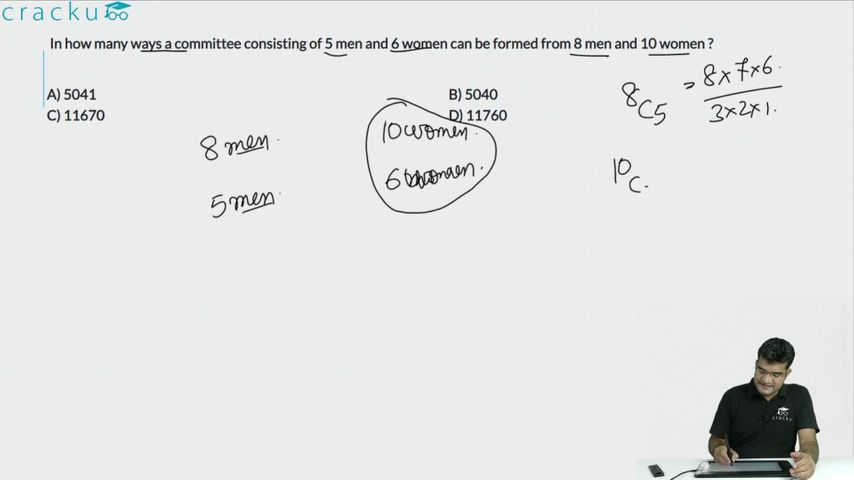Question 1

# In how many ways a committee consisting of 5 men and 6 women can be formed from 8 men and 10 women ?

Solution

Number of ways a committee consisting of 5 men and 6 women can be formed from 8 men and 10 women

= $$C^{10}_6\times C^8_5$$

= $$C^{10}_4\times C^8_3$$

= $$\frac{10\times9\times8\times7}{1\times2\times3\times4}\times\frac{8\times7\times6}{1\times2\times3}$$

= $$(10\times3\times7)\times(8\times7)$$

= $$210\times56=11760$$

=> Ans - (D)

### View Video Solution